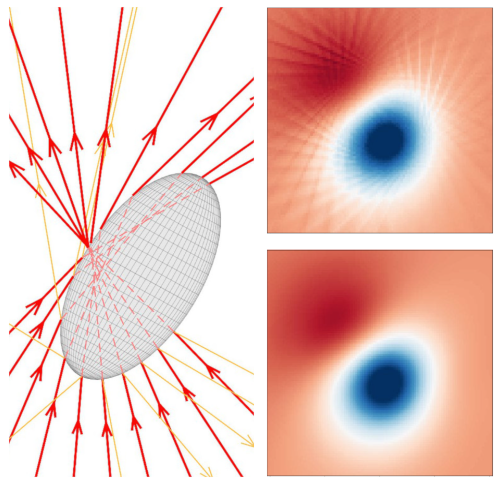## Faster and more accurate geometrical-optics optical force calculation using neural networks published in ACS PhotonicsFocused rays scattered by an ellipsoidal particles (left). Optical torque along y calculated in the x-y plane using ray scattering with a grid of 1600 rays (up, right) and using a trained neural network (down, right). (Image by the Authors of the manuscript.)Faster and more accurate geometrical-optics optical force calculation using neural networks
David Bronte Ciriza, Alessandro Magazzù, Agnese Callegari, Gunther Barbosa, Antonio A. R. Neves, Maria A. Iatì, Giovanni Volpe, Onofrio M. Maragò
ACS Photonics, 2022
doi: 10.1021/acsphotonics.2c01565
arXiv: 2209.04032

Optical forces are often calculated by discretizing the trapping light beam into a set of rays and using geometrical optics to compute the exchange of momentum. However, the number of rays sets a trade-off between calculation speed and accuracy. Here, we show that using neural networks permits one to overcome this limitation, obtaining not only faster but also more accurate simulations. We demonstrate this using an optically trapped spherical particle for which we obtain an analytical solution to use as ground truth. Then, we take advantage of the acceleration provided by neural networks to study the dynamics of an ellipsoidal particle in a double trap, which would be computationally impossible otherwise.